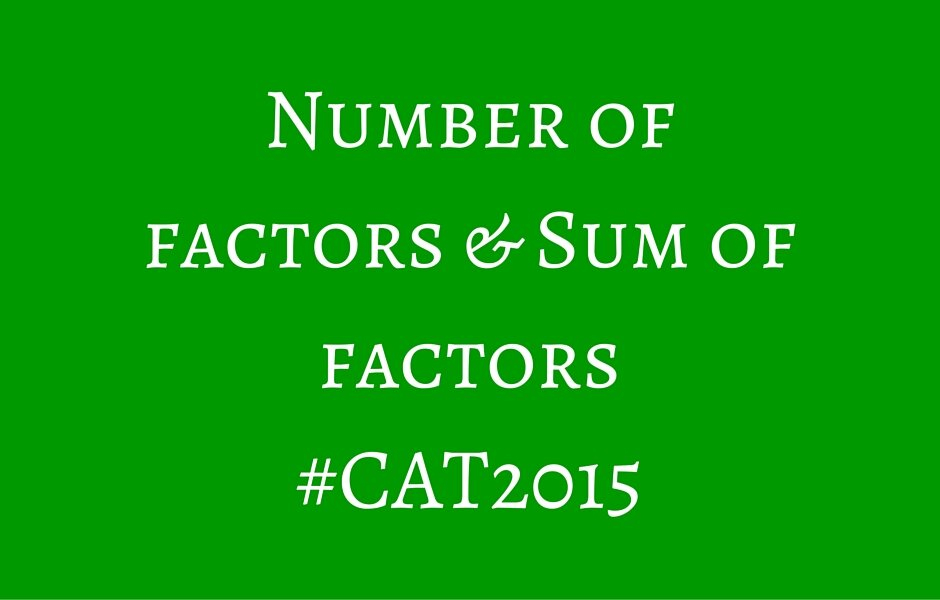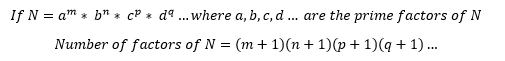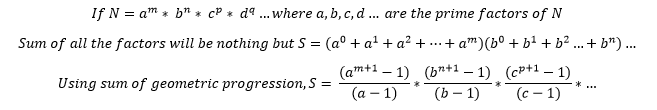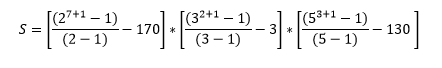Select PageThis is a concept known to a lot of aspirants but very few tend to think beyond the basic formulae that are present. The questions are generally in an almost unrecognizable format or are a bit different than the textbook questions one has ‘prepared’ for. In this article, I will try to explore the concepts and solve a few tricky examples dealing with number of factors and sum of factors.

Number of factors

The basic premise is simple; every number will have a certain number of integral factors. For smaller numbers, we can manually calculate the same but for larger numbers, it becomes difficult to do the same. So, we look for a mathematical solution to ensure that we have to put in minimal effort while solving such questions. Let’s look at a few examples and try to figure out the logic behind the formula.

 Number Representation Factors Number of factors 24 23 * 31 (1,2,3,4,6,8,12,24) 8 36 22 * 32 (1,2,3,4,6,9,12,18,36) 9 45 32 * 51 (1,3,5,9,15,45) 6 80 24 * 51 (1,2,4,5,8,10,16,20,40,80) 10 100 22 * 52 (1,2,4,5,10,20,25,50,100) 9

To cut the long story short, number of factors of a particular integer (N) is given by:A lot of students know the formula but are not aware of the intricacies of the same that leads to half knowledge while attempting questions leading to wastage of precious time during the test. To understand the formula, we have to know why we add 1 to each power. When we say that am is a factor of a number N, we understand that each of am, am-1, am-2… a2, a1, a0 will also be a factor of N. Simply speaking, we have to count all the factors from 0 till m and that’s why we add 1 to each of the factors. Now, say for example that we require all the factors that do not have a3 in them, then we will ignore all the factors starting from a3 till am and consider only a0, a1 and a2. A lot of questions are formed around this concept and if one isn’t careful, it can lead to loss of easy marks.

A few important things about the number of factors:

• The highest prime factor of a number will always be less than or equal to the square root of the number
• Squares of prime numbers have exactly 3 factors
• If a number has an odd number of factors, it has to be a perfect square, conversely, if a number does not have an odd number of factors, it cannot be a perfect square
• The number can be formed by multiplying two factors on either side of the square root of the number
• A prime number will have exactly two factors, 1 and the number itself
• In special cases and question types, you might have to consider negative factors as well; generally though, the questions would involve positive factors of a number only
• Every number will have at least two factors: 1 and the number itself
• Any prime number greater than 3 can be expressed in the form on (6k + 1)

Sum of factors

A slightly more complicated concept involves finding the sum of factors. Again, it is an extension of the number of factors and the properties of a geometric progression and extremely derivable.which is essentially the sum of the factors. Again, using a bit of logic, we can figure out that if a particular factor is not required, we can simply eliminate it from the required bracket and leave the rest as it is and calculate the answer.

Let’s look at a few tricky questions from this topic.

You are a mathematician participating in the final round of the X-Factor Championship. As the name suggests, the final round involves you getting a random number of which, you have to find the number of factors that are perfect squares. You nervously make your way on to the stage, knowing that your opponent has missed his chance and that the right answer will ensure your victory. The random number generator spits out the number 144000. What would be the number you would come up with to win the X-Factor Championship?

If you can look beyond the theatrics, it simply means that you need to find the number of factors of 144000 that are perfect squares. Factorizing 144000 you get:

144000 = 27 * 32 * 53

Now, for a factor to be a perfect square, it should have all even powers. So, the powers of 2 could be either (0,2,4 or 6) that of 3 could be (0 or 2) and that of 5 could be (0 or 2). So, there is a total of 4*2*2 ie. 16 factors that would be perfect squares.

Continuing the X-Factor Championship story forward, the organizers could not believe it that you had given the correct answer and so, they change the rules at the last moment and ask you to prove your mettle by calculating the sum of all the factors in the next 5 minutes. What would you answer knowing that this is probably your last shot at glory?

Again, you have to eliminate the useless factors and calculate for the remaining ones and apply the sum of factors formula. The useless parts in each bracket would be: (21+23+25+27=170), (31=3) and (51+53=130)

So, we get the sum of required factors to be:Which will be equal to 85*10*26 = 22100

There is an interesting game show called What’s The Factor? designed to test your calculation skills. In one of the rounds, you are supposed to find the number of factors of 144000 that are multiples of 36. What would be your answer?

The number has to be a multiple of 36 = 22 * 32

So, the minimum powers of 2 and 3 have to be 2 each and then we can have a list of numbers. So, the total number of factors that are multiples of 36 would be = 6*1*4 = 24 factors.

A question for practice:

There is a row of piggy banks kept at an exhibition to collect funds. Also, there are 100 mathematicians who are attending the exhibition. The first mathematician puts Rs. 1 in each of the piggy banks, the second mathematician puts Rs. 2 into the second, fourth, sixth, eight piggy banks till 100, the third mathematician puts Rs. 3 into the third, sixth, ninth, twelfth piggy banks, the fourth puts Rs. 4 into the fourth, eighth, twelfth piggy bank and so on. Which among the following has the largest contribution at the end of the event?

(a) 100         (b) 64           (c) 90           (d) 96

You can go through the entire series of 75 days to CAT 2015 to check out more such concept and strategy based articles.

All the best!

error: Content is protected !!